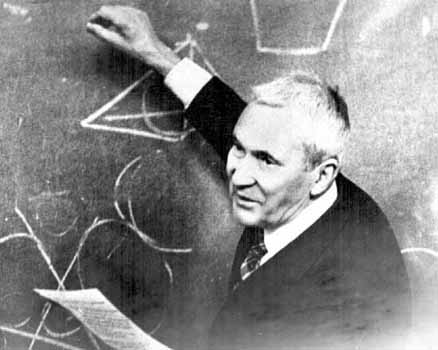# What is Kolmogorov Complexity?## The Basic Idea

I’ve been reading about Kolmogorov complexity, with the aim of understanding certain metamathematical results (including general incompleteness). I seem to be on the verge of understanding specifically Chaitin’s reformulation of Gödel’s results using the Berry paradox.

First, before getting to the paradox let’s define Kolmogorov complexity (or Kolmogorov-Chaitin-Solomonoff complexity; so-called after the 3 individauals who independently discovered it). Assuming a given string (or string rendering of a particular problem), the Kolmogorov complexity of that string is defined as the size of the smallest program that is needed to generate that string. For example (I’m using some of the examples from here), let’s assume that we have the following two strings of size $n=8$:

$$x = 01010101 \\ x’ = 11101001$$

In the first case, a reasonably compact Python program for generating $x$ is the following:

print("01"*4)


which, excluding the Python parts ",print, (,), * and the prefix "01" (since these wil stay constant for all such patterns longer than $n=8$), has a size of $4$ (or $n/2$). In bits this is equivalent to the following (using the laws of number-bit conversion; this part about the conversion tripped me up a bit at first, but is essential for the result in the next Section): $$\left\lfloor \log_{2}(4) \right\rfloor + c$$ where $c (\ge 1)$ is the constant that covers the programming language parts and the constant pattern 01.

Now what about the second string $x’$? Clearly, this pattern is more complex, in that sense that it is difficult to come up with a more compact program than the following:

print("11101001")


which simply returns the total pattern "11101001", and is therefore of size equal to the size of the input (for now, forget the point about the $log_{2}$ bit conversion).

With these ideas in mind, we can then quantify the notion of a random string as one whose smallest program is greater than or equal to the size of the input string plus this constant $c$ (in other words, we cannot find a program smaller than the one that simply encodes and returns the full string). We can further define the set $R$ of all random strings as follows (where $K(x)$ stands for Kolmogorov complexity):

$$R = \bigg\{ x \mid K(x) \ge | x | \bigg\}$$

I find this definition of randomness to be very satisfying. In the simplest terms, it says that a string is random if we cannot come up with a clever pattern to describe or generate it (alternatively, you can think about a random string as one that we cannot compress down to something smaller; in other presentations, $R$ is sometimes referred to as the set of uncompressable strings). To me it is very easy to imagine many such strings, though this notion of randomness turns out to be fundamentally problematic, as described next.

## The Result

Now we can ask the question: can we come up with a general algorithm to find this set $R$ and determine if a given $x$ is random? Somewhat shockingly, it turns out we can’t according to the following result (it took me some time to find a readable proof; this one is based on this blog post already cited above, these notes here and this wonderful textbook, which I’m still working through):

Theorem $R$ is not decidable.

Proof. Let’s imagine a computer program $M$ (e.g., a Turing machine, Python program, whatever; as it turns out, the choice of programming language is not so important), that computes $R$. If such a program exists, then we can use it for storing all random strings in sorted order (we can further represent this program $M$ as a string of size $| M |^{\texttt{bits}}$, where $| \cdot |^{\texttt{bits}}$ is the size of the program string in bits; this exploits the fact that we can encode Turing machines as binary strings).

To find if a particular string $x \in R$ (where $|x|=n$), we can then use another program $M’$ that will simply do a look up on $R$ for all strings of size $n$ and return true if it finds the input $x$. Imagine that we represent this latter program as a string $s_{n}$ built from a string rendering of $M’$ (of size $| M’ |^{\texttt{bits}}$) concatenated with the number $n$ (where again, the bit representation of $| n |^{\texttt{bits}}$ is approximately $log_{2}(n)$, as discussed above). In other words: $$| s_{n} |^{\texttt{bits}} = | M’ |^{\texttt{bits}} + | n |^{\texttt{bits}}$$ The problem is that according to our definition of randomness, the following must hold: $$K(s_{n}) \ge n$$ whereas $s_{n}$ will have a length of $c + \log_{2} n$ (where $M,M’$ get stuffed into our constant $c$). Therefore, we have the following: $$n \le \log_{2}(n) + c$$ which cannot hold for most $n$. Therefore $R$ cannot be computed in the general case. □

As once remarked by Marvin Minsky, Kolmogorov complexity therefore has the fatal flaw that, in the general case, it is not possible to compute exactly what the theory is designed to compute!

## Why Does This Happen?

As mentioned at the onset, this result can be used to derive the famous Gödel incompleteness results (see Chaitin’s paper for more details). The source of these results is the so-called Berry Paradox, which was first published by Bertrand Russell but named after an obscure Oxford University librarian who first posed the paradox to Russell (as Chaitin describes, it can be viewed as a variant of the liar paradox that Gödel relied on to prove his famous results). The paradox can be understood by thinking about the following description of a number:

The smallest positive integer than cannot be described in less than 1 billion words in English.

While we can imagine the particular number being described, the paradox is that we just described such a number using far less than 1 billion English words (more exactly, we used only 16 English words!). This is the essense of the proof above, which involves this $\log_{2}$ bit compression trick.